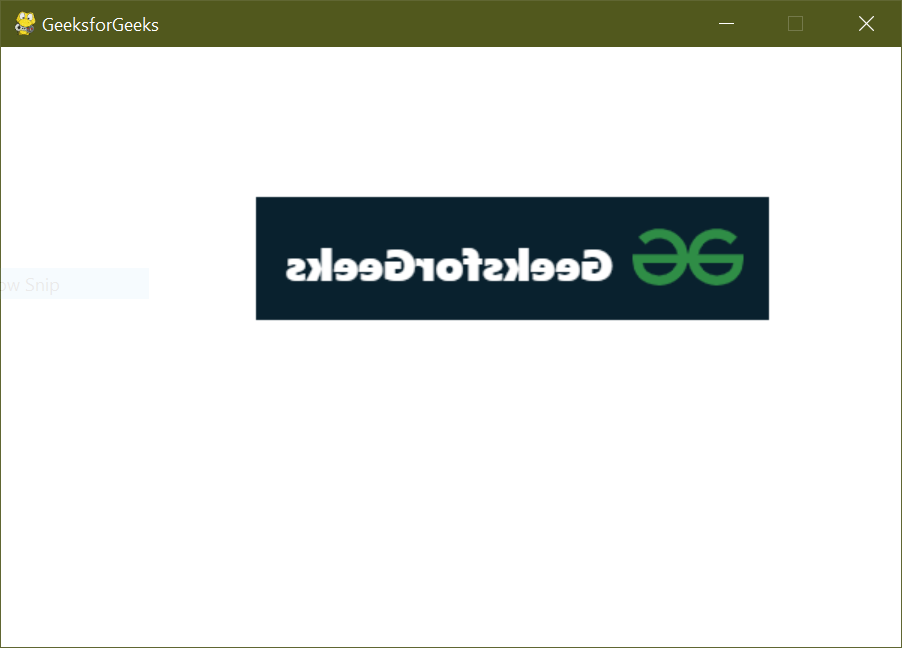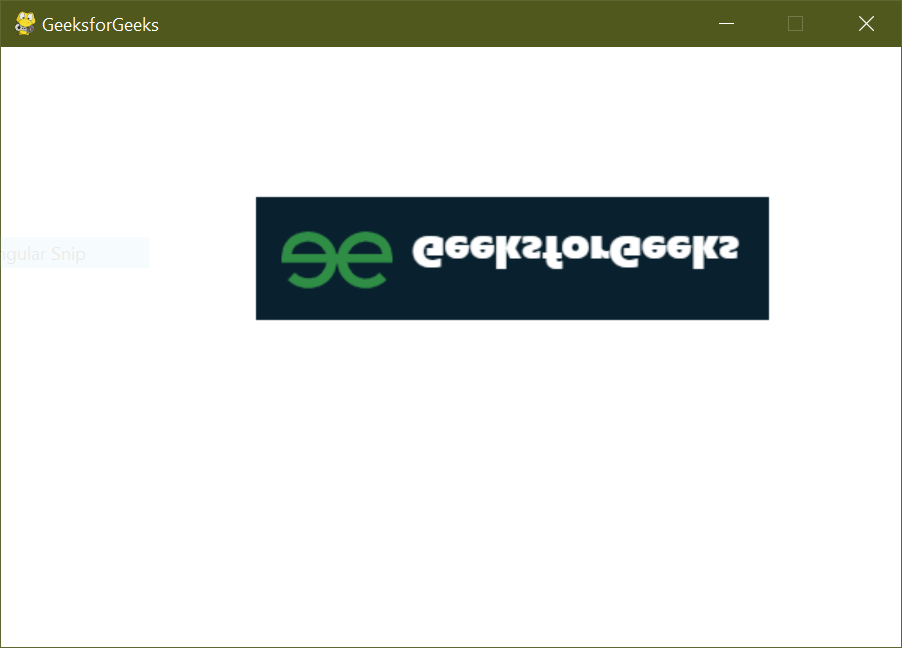Related Articles

# Pygame – Flip the image

• Last Updated : 30 Jun, 2021

In this article, we are going to see how images can be flipped using Pygame.

To flip the image we need to use pygame.transform.flip(Surface, xbool, ybool) method which is called to flip the image in vertical direction or horizontal direction according to our needs.

Syntax:

pygame.transform.flip(Surface, xbool, ybool)

### Flip the image in vertical direction

In this, we have to flip the image in a vertical direction. We will use pygame.transform.flip() to display the image in vertical. Pass xbool as True and ybool as False, so that the image is flipped vertically.

Input used:## Python3

 `# import pygame and sys``import` `pygame``import` `sys`` ` `from` `pygame.``locals` `import` `*`` ` `# pygame.init() will initialize all``# imported module``pygame.init()``pygame.display.set_caption(``'GeeksforGeeks'``)`` ` `# screen size will display on screen``screen ``=` `pygame.display.set_mode((``600``, ``400``), ``0``, ``32``)`` ` `# pygame.image.load() will return the``# object that has image``img ``=` `pygame.image.load(``'image.png'``)`` ` `while` `True``:``     ` `    ``# Background color``    ``screen.fill((``255``, ``255``, ``255``))``     ` `    ``# image copy``    ``img_copy ``=` `img.copy()``     ` `    ``# pygame.transform.flip() will flip the image``    ``img_with_flip ``=` `pygame.transform.flip(img_copy, ``True``, ``False``)``     ` `    ``# surface.blit() function draws a source ``    ``# Surface onto this Surface.``    ``screen.blit(img_with_flip, (``50` `+` `1` `*` `120``, ``100``))``     ` `    ``# event listener to quit screen``    ``for` `event ``in` `pygame.event.get():``        ``if` `event.``type` `=``=` `QUIT:``            ``pygame.quit()``            ``sys.exit()`` ` `    ``# update the frame per second``    ``pygame.display.update()`

Output### Flip the image in horizontal direction

In this we have to flip the image in horizontal direction. For this xbool is passed as False and ybool as True, to flip it horizontally.

Program:

## Python3

 `# import pygame and sys``import` `pygame``import` `sys`` ` `from` `pygame.``locals` `import` `*`` ` `# pygame.init() will initialize all ``# imported module``pygame.init()``pygame.display.set_caption(``'GeeksforGeeks'``)`` ` `# screen size will display on screen``screen ``=` `pygame.display.set_mode((``600``, ``400``), ``0``, ``32``)`` ` `# pygame.image.load() will return the``# object that has image``img ``=` `pygame.image.load(``'image.png'``)`` ` `while` `True``:``     ` `    ``# Background color``    ``screen.fill((``255``, ``255``, ``255``))``     ` `    ``# image copy``    ``img_copy ``=` `img.copy()``     ` `    ``# pygame.transform.flip() will flip the image``    ``img_with_flip ``=` `pygame.transform.flip(img_copy, ``False``, ``True``)``     ` `    ``# surface.blit() function draws a source ``    ``# Surface onto this Surface.``    ``screen.blit(img_with_flip, (``50` `+` `1` `*` `120``, ``100``))``     ` `    ``# event listener to quit screen``    ``for` `event ``in` `pygame.event.get():``        ``if` `event.``type` `=``=` `QUIT:``            ``pygame.quit()``            ``sys.exit()`` ` `    ``# update the frame per second``    ``pygame.display.update()`

OutputAttention geek! Strengthen your foundations with the Python Programming Foundation Course and learn the basics.

To begin with, your interview preparations Enhance your Data Structures concepts with the Python DS Course. And to begin with your Machine Learning Journey, join the Machine Learning – Basic Level Course

My Personal Notes arrow_drop_up